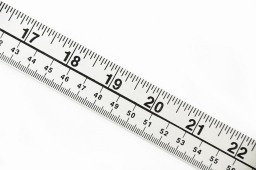# A length

A length of 10/15 meters is cut from an electric wire whose length is 13/15 meters. How long is the remaining piece of wire?

r =  1/5 m

### Step-by-step explanation:Did you find an error or inaccuracy? Feel free to write us. Thank you!

Tips for related online calculators
Need help calculating sum, simplifying, or multiplying fractions? Try our fraction calculator.
Do you want to convert length units?